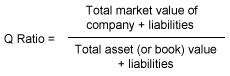## Definition of Tobin's q ratio

Tobin's q, or the q ratio, is the ratio of the market value of a company's assets (as measured by the market value of its outstanding stock and debt) divided by the replacement cost of the company's assets (book value).

## Calculating a q ratioQ ratios can also be used for calculating a stock market valuation. In this case the aggregate of the stock market values for companies in a given stock market or index is divided by the aggregate of the replacement value of those companies' assets.

## q ratio in the news

In September 2013 an FT columnist examined two competing ways of analysing stock market valuations – the q ratio and Cape. Although the two methods used very different calculations, both pointed to the fact that the US stock market was significantly overvalued leading some to question the accuracy of both methods.

## FT Articles & Analysis

No articles are associated with this term